﻿ A Markov Chain Application on the Levels of the Bloom’s Taxonomy of Learning ObjectivesPublications are Open
Access in this journal
Article Versions
Export Article
• Normal Style
• MLA Style
• APA Style
• Chicago Style
Research Article
Open Access Peer-reviewed

### A Markov Chain Application on the Levels of the Bloom’s Taxonomy of Learning Objectives

Michael Gr. Voskoglou
American Journal of Educational Research. 2019, 7(3), 294-298. DOI: 10.12691/education-7-3-15
Received November 18, 2018; Revised January 03, 2019; Accepted March 28, 2019

### Abstract

A Markov Chain is introduced on the levels of the Bloom’s Taxonomy and a measure for evaluating student learning skills is obtained by applying basic principles of the related theory. A classroom application is also presented illustrating the usefulness of this approach in practice. The Bloom’s Taxonomy, which has been applied in the USA and in other countries by generations of teachers and college instructors in the teaching process, refers to a classification of the different learning objectives serving as a way of distinguishing the fundamental questions within the educational system.

### 1. Introduction

The knowledge that students have about various concepts is usually imperfect characterized by a different degree of depth. On the other hand, from the teacher’s point of view there exists frequently an uncertainty about the acquisition of a subject matter by students. All these gave us the hint in earlier works to introduce principles of fuzzy logic for a more effective description of the process of learning and for the assessment of student learning skills [ 1, 2: Chapters 6, 7], etc.

In the paper at hands we follow a different approach to deal with the uncertainty appearing in learning situations. Namely, we introduce a Markov Chain (MC) on the levels of the Bloom’s Taxonomy (BT) of learning objectives and applying basic principles of the corresponding theory we obtain a measure for evaluating student learning skills. The BT, which has been applied in the USA 3 and in other countries by generations of teachers and college instructors in the teaching process, refers to a classification of the different learning objectives serving as a way of distinguishing the fundamental questions within the educational system.

The rest of the paper is formulated as follows. In Section 2 the central ideas of the BT are exposed and its levels are analyzed. In Section 3 the MC model on the levels of BT is constructed, while in Section classroom application is presented illustrating its usefulness in practice. The paper closes with the final conclusions and some hints for future research contained in Section 5.

### 2. The Bloom’s Taxonomy

In 1956 Benjamin Bloom with collaborators Max Englehart, Edward Furst, Walter Hill, and David Krathwohl published a framework for categorizing educational goals, the Taxonomy of Educational Objectives 4. Although named after Bloom, the publication of the taxonomy followed a series of conferences from 1949 to 1953, which were designed to improve communication between educators on the design of curricula and examinations.

BT divides educational objectives into three domains: cognitive, affective and psychomotor, sometimes loosely described as "knowing/head", "feeling/heart" and "doing/hands" respectively. The volume published in 1956 4 and the revision followed in 2000 5 concern the cognitive domain, while a second volume was published in 1965 on the affective domain. A third volume was planned on the psychomotor domain, but it was never published. However, other authors published their own taxonomies on the last domain. More details can be found in 6.

The revised version of the taxonomy 5 was created by Lorin Anderson, former student of Bloom. Since the taxonomy reflects different forms of thinking and thinking is an active process, in the revised version the names of its six major levels were changed from noun to verb forms.

The six major levels of the revised taxonomy are presented in Figure 1, taken from 6.

• Figure 1. The six major levels of the Bloom’s taxonomy

The above six levels in the taxonomy, moving through the lowest order processes to the highest, could be described as follows :

Ÿ Knowing-Remembering: Retrieving, recognizing, and recalling relevant knowledge from long-term memory. e.g. find out, learn terms, facts, methods, procedures, concepts

Ÿ Organizing-Understanding: Constructing meaning from oral, written, and graphic messages through interpreting, exemplifying, classifying, summarizing, inferring, comparing, and explaining. Understand uses and implications of terms, facts, methods, procedures, concepts.

Ÿ Applying: Carrying out or using a procedure through executing, or implementing. Make use of, apply practice theory, solve problems and use information in new situations.

Ÿ Analyzing: Breaking material into constituent parts, determining how the parts relate to one another and to an overall structure or purpose through differentiating, organizing, and attributing. Take concepts apart, break them down, analyze structure, recognize assumptions and poor logic, evaluate relevancy.

Ÿ Generating-Evaluating: Making judgments based on criteria and standards through checking and critiquing. Set standards, judge using standards, evidence, rubrics, accept or reject on basis of criteria.

Ÿ Integrating - Creating: Putting elements together to form a coherent or functional whole; reorganizing elements into a new pattern or structure through generating, planning, or producing. Put things together; bring together various parts; write theme, present speech, plan experiment, put information together in a new & creative way.

The last three levels -analyzing, evaluating and creating – are parallel to each other characterized by successive transitions from one to another. It is obvious that the acquisition of the three higher levels of the BT helps the students to become better problem solvers.

Teaching a topic, the instructor should arrange the class work in order to synchronize it with the six steps of BT. The typical questions for evaluating the student achievement at the corresponding level are the following:

Knowing questions focus on clarifying, recalling, naming, and listing: Which illustrates...? Write... in standard form.... What is the correct way to write the number of... in word form?

Organizing questions focus on arranging information, comparing similarities/ differences, classifying, and sequencing: Which shows... in order from...? What is the order...? Which is the difference between a... and a...? Which is the same as...? Express... as a...?

Applying questions focus on prior knowledge to solve a problem: What was the total...? What is the value of...? How many... would be needed for...? Solve....Add/subtract....Find....Evaluate....Estimate....., graph.

Analyzing questions focus on examining parts, identifying attributes/ relationships /patterns, and main idea: Which tells...? If the pattern continues....which could...? What rule explains/completes... this pattern? What is/are missing? What is the best estimate for...? Which shows...? What is the effect of...?

Generating questions focus on producing new information, inferring, predicting, and elaborating with details: What number does... stand for? What is the probability...? What are the chances...? What effect...?

Integrating questions focus on connecting/combining/summarizing information and restructuring existing information to incorporate new information: How many different...? What happens to... when...? What is the significance of...? How many different combinations...? Find the number of..., ..., and ... in the figure below.

Evaluating questions focus on reasonableness and quality of ideas, criteria for making judgments and confirming accuracy of claims: Which most accurately...? Which is correct? Which statement about... is true? What are the chances...? Which would best...? Which would... the same...? Which statement is sufficient to proven...?

BT serves as the backbone of many teaching philosophies, in particular those that lean more towards skills rather than content. The emphasis on higher-order thinking inherent in such philosophies is based on the top levels of the taxonomy including analysis, evaluation, synthesis and creation. BT can be used as a teaching tool to help balance assessment and evaluative questions in class, assignments and texts to ensure all orders of thinking are exercised in student’s learning.

### 3. The Markov Chain Model

A MC is a stochastic process that moves in a sequence of steps (phases) through a set of states and has a “one-step memory”. This means that the probability of entering a certain state in a certain step, known as the transition probability between steps, depends on the state occupied in the previous step and not in older steps. This is known as the Markov property. However, for being able to model as many real life situations as possible by using MCs, one could accept in practice that the transition probability, although it may not be completely independent of previous steps, it mainly depends on the state occupied in the previous step 7.

The basic concepts of MCs were introduced by A. Markov in 1907 on coding literal texts. Since then the MC theory was developed by a number of leading mathematicians, such as A. Kolmogorov, W. Feller, etc. However, only from the 1960’s the importance of this theory to the natural, social and most of the applied sciences has been recognized ( 7, 8, 9, etc.).

When the set of states of a MC is a finite set, then we speak about a finite MC. For general facts on finite MCs we refer to the book 10.

Here, for studying mathematically the learner’s movements among the first three levels of the BT (Figure 1), we introduce a finite MC with states the levels S1 = Remember, S2 = Understand, S3 = Apply and S4 = Analyze/Evaluate/Create. For reasons of simplicity, the three higher levels of the BT, which are parallel to each other, have been joined in a single state of the MC. The flow-diagram of this chain is represented in Figure 2, where the arrows returning to the same state correspond to cases where the learner, being in a level of the BT, does not succeed at a certain phase to proceed to the next level thus remaining in the same one.

• Figure 2. The flow-diagram of the MC

Denote by pij the transition probability from state Si to state Sj, i, j = 1, 2, 3, 4. Then the matrix A= [pij] is called the transition matrix of the MC. Taking into account the flow-diagram of Figure 2 one concludes that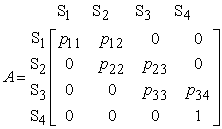(1)

Since the transition from a state to some other state is the certain event, we have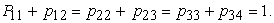(2)

A state of a MC is called absorbing if, once entered, it cannot be left. Further a MC is said to be an absorbing MC (AMC) if it has at least one absorbing state and if from every state it is possible to reach an absorbing state, not necessarily in one step. Obviously the present MC is an AMC with S4 its unique absorbing state and S1 its starting state.

Applying the standard theory of the AMCs ( 10, Chapter 3) we bring the transition matrix A to its canonical form A* by listing the absorbing state first and then we make a partition of A* as follows: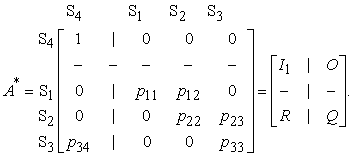(3)

In the above partition I1 is the 1 X 1 unitary matrix, O is a 1 X 3 zero matrix, R is the 3 X 1 transition matrix from the non-absorbing states to the absorbing state and Q is the 3 X 3 transition matrix between the three non absorbing states. Then, if I3 denotes the 3 X 3 unitary matrix, we have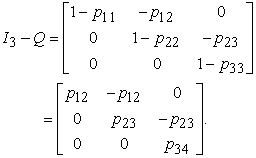(4)

This matrix is in all AMCs invertible 11. The fundamental matrix N of the AMC is defined to be the inverse matrix of I3 – Q. Therefore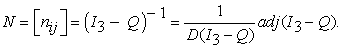(5)

In equation (5) adj (I3 – Q) denotes the adjoin matrix of I3 – Q and D (I3 – Q) denotes the determinant of I3 Q ( 12, Section 2.4). It is recalled that the adjoin matrix of I3 – Q is the matrix of the algebraic complements of the transpose matrix of I2 – Q, which is obtained by turning the rows of I2 – Q to columns and vice versa.. Replacing the matrix I3 – Q from (4) to (5) and making the corresponding calculations one finds that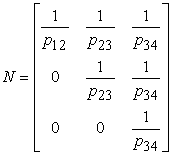(6)

It is well known ( 10, Chapter 3) that the element nij of the fundamental matrix N gives the mean number of times in state Si before the absorption, when the starting state of the AMC is Sj , where Si and Sj are non absorbing states. In our case, since S1 is the starting state of the MC, it becomes evident that the mean number of its steps before the absorption is given by the sum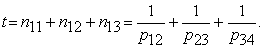(7)

It is logical to accept that the greater is the value of t, the more the learner’s difficulties for the acquisition of the first three levels of the BT. On the other hand, the total time spent is another factor, apart for t, indicating the learner’s difficulties. However, in case of learning a subject matter in the classroom, the time available is usually prefixed, which means that in this case t could be considered as a measure of the student difficulties for the acquisition of the first three steps of the BT.

### 4. A Classroom application

The following application was performed with subjects the students of two different departments (30 students in each department) of the School of Technological Applications (prospective engineers) of the Graduate Technological Educational Institute (T. E. I.) of Western Greece attending the common course “Mathematics I” of their first term of studies and having the same instructor. This course involves an introductory module repeating and extending the students’ knowledge from secondary education about the real numbers. After the module was taught the instructor, in order to investigate the students’ progress according to the principles of the BT, he asked them to answer in the class the questions of the following test, which is divided in three parts corresponding to the first three levels of the taxonomy. The answers were given in writing and the time allowed was the same for both departments.

Part I (Knowing – Remembering)

Ÿ Give the definitions and examples of a periodic decimal and of an irrational number in the form of an infinite decimal.

Part II (Organizing)

Ÿ Compare the set of all fractions with the set of periodic decimals and the set of irrational numbers with the set of all roots (of any order) that have no exact values. Justify your answers.

Part III (Applying)

Ÿ Which of the following are natural numbers, integers, rational, irrational and real numbers?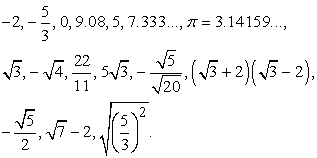The results of the test were the following:

Part I: 27 satisfactory answers for the first and 25 for the second department.

Part II: 25 satisfactory answers for the first and 25 for the second department.

Part III: 22 satisfactory answers for the first and 24 for the second department.

Going back to the MC model of the previous section, the above outcomes determine the following values for the transition probabilities:

First department: p11 =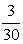, p12 =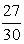, p22 =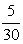, p23=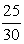, p33 =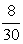, p34 =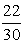.

Second department: p11 =, p12 =, p22 =, p23=, p33 =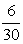, p34 =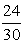.

Replacing the above values to equation (7) and making the corresponding numerical calculations one finds that t =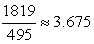for the first and t =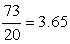for the second department. This means that the students of the first department faced slightly more difficulties for the acquisition of the first three levels of the BT than the students of the second department did.

### 5. Discussion and Conclusions

MC theory is a smart combination of Linear Algebra and Probability that can be effectively used for modeling uncertain situations and making forecasts for their evolution. In particular, human reasoning is characterized by a high degree of uncertainty and therefore MCs is a useful tool for studying human cognitive activities, like learning, problem-solving, decision-making, etc. (e.g. see 2, Chapter 3).

In the present work we introduced a finite AMC on the first three levels of the BT and applying principles of the relevant theory we have obtained a measure of the learner’s difficulties for the acquisition of those levels.

Concerning the remaining three higher levels of the BT, the application of an analogous approach for their study seems to be difficult, since they are parallel characterized by successive transitions from one to another level. Consequently, the calculation of the corresponding transition probabilities looks to be more complicated and further research is needed on it. In addition, a possible increase of the states of the MC from four to six makes harder the development of the corresponding mathematical model involving laborious calculations. Note that, the resulting in this case MC is an ergodic one, where it is possible to move from any state to another state, not necessarily in one step. This means that results from the theory of the ergodic MCs ( 10, Chapter 4) and not of the ABCs must be applied in this case for the development of the analogous mathematical model.

Other mathematical tools like fuzzy logic and its generalizations have been also used by the present author for studying human – machine (computers) activities ( 2, Chapters 5-7, 13, 14, etc.). Each one of those tools has its own strengths and disadvantages. A combination of MC theory and fuzzy logic for obtaining better results is one of the main priorities of our future research on this field.

### References

  Voskoglou, M. Gr., “Fuzziness or Probability in the Process of Learning: A General Question Illustrated by Examples from Teaching Mathematics”, The Journal of Fuzzy Mathematics, 17(3), 679-686, 2009. In article  Voskoglou, M. Gr., Finite Markov Chain and Fuzzy Logic Assessment Models: Emerging Research and Opportunities, Createspace.com – Amazon, Columbia, SC, USA, 2017. In article  Bloom, B. S., Rehage, K. J., Anderson, L. W. Sosniak, L. A. (Eds.), “Bloom's taxonomy: A forty-year retrospective”, Yearbook of the National Society for the Study of Education (Chicago: National Society for the Study of Education), 93 (2), 1994. In article  Bloom, B. S., Engelhart, M. D., Furst, E. J., Hill, W. H., Krathwohl, D. R., Taxonomy of educational objectives: The classification of educational goals, Handbook I: Cognitive domain, David McKay Company, New York ,1956. In article  Anderson, L. W., (Eds.), A taxonomy for learning, teaching, and assessing: A revision of Bloom's taxonomy of educational objectives, Allyn and Bacon, Boston, 2000. In article  Wikipedia, Bloom’s Taxonomy, retrieved from http://en.wikipedia.org/wiki/Bloom’s_taxonomy, on May, 2018. In article  Kemeny, J. G. & Snell, J. L., Mathematical Models in the Social Sciences, Ginn and Company, New York, USA, 1963. In article  Suppes, p. & Atkinson, R.S., Markov Learning Models for Multiperson Interactions, Stanford University Press, Stanford, California, 1960. In article  Bartholomew, D.J., Stochastic Models for Social Processes, J. Wiley and Sons, London, 1973. In article  Kemeny, J. G. & Snell J. L., Finite Markov Chains, Springer - Verlag, New York, USA, 1976. In article  Voskoglou, M. Gr. & Perdikaris, S. C., A Markov chain model in problem- solving, International Journal of Mathematical Education in Science and. Technology, 22, 909-914, 1991. In article View Article  Morris, A. O., An Introduction to Linear Algebra, Van Nostrand Beinhold Company Ltd., Berkshire, England, 1978. In article  Voskoglou, M. Gr., Use of TFNs and TpFNs for Evaluating the Effectiveness of CBR Systems, International Journal of Fuzzy Systems and Advanced Applications, 4, 1-7, 2017. In article  Voskoglou, M. Gr., Application of Grey Numbers to Assessment of the Understanding of the Graphical Representation of the Derivative, American Journal of Educational Research, 5(11), 1167-1171, 2017.. In article View ArticleThis work is licensed under a Creative Commons Attribution 4.0 International License. To view a copy of this license, visit http://creativecommons.org/licenses/by/4.0/